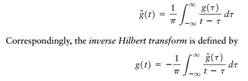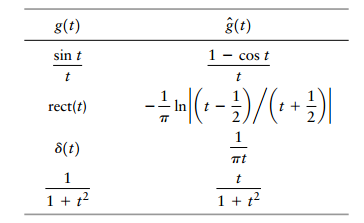Create an Account

Home / Questions / A Hilbert transformer may be viewed as a device whose transfer function exhibits the follo...

A Hilbert transformer may be viewed as a device whose transfer function exhibits the following characteristics a The amplitude response is unity for all positive and negative frequencies

A Hilbert transformer may be viewed as a device whose transfer function exhibits the following characteristics:

(a) The amplitude response is unity for all positive and negative frequencies.

(b) The phase response is for negative frequencies and for positive frequencies. Starting with the definition of the Hilbert transform given in Problem 2.52, demonstrate the frequency-domain description embodied in parts (a) and (b).

Problem 2.52

The Hilbert transform of a Fourier transformable signal g(t) is defined byUsing these two formulas, derive the following set of Hilbert-transform pairs:View less »

Jul 31 2020 View more View LessSubscribe To Get Solution